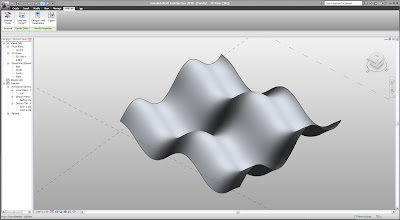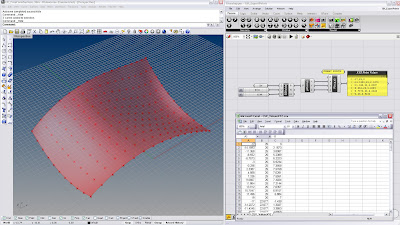## Tuesday, January 5, 2010

### Revit API: Mathematical Form-Making

In addition to my continued exploration with Grasshopper, I will begin doing posts relating to my recent interest with the Revit API.... and as my previous post suggests, these interests are not necessarily going to be "exclusive" from one another....

Below are some examples of making some parametric surfaces using a simple API script. The kinds of surfaces you are able to create is limited for two reasons:
1. There are only about 4 ways to create a surface (Loft, extrude, sweep, revolve)...
A surface from a point cloud or from 4-points would be very handy, Autodesk!
2. Revit doesn't seem to "like" self-intersecting surfaces very much... and even sometimes mistakes 'closed' shapes or shapes where edges touch for self-intersection....so no Kleins or Catalans, booo!

Wave SurfaceSample Code (Wave surface):
Dim ref_ar_ar As ReferenceArrayArray = New ReferenceArrayArray()
Dim XYZ As Autodesk.Revit.Geometry.XYZ

For u As Double = 0 To 4 * Math.PI Step Math.PI / 4
Dim rfptsarr As ReferencePointArray = New ReferencePointArray()
For v As Double = 0 To 4 * Math.PI Step Math.PI / 4

Dim refpt As Autodesk.Revit.Elements.ReferencePoint

Dim x As Double
Dim y As Double
Dim z As Double

x = 10 * u
y = 10 * v
z = 10 * Math.Cos(u) + 10 * Math.Sin(v)

XYZ = revit_app.Create.NewXYZ(x, y, z)
refpt = revit_doc.FamilyCreate.NewReferencePoint(XYZ)

rfptsarr.Append(refpt)
Next
Dim crv As CurveByPoints = revit_doc.FamilyCreate.NewCurveByPoints(rfptsarr)
Dim ref_ar As ReferenceArray = New ReferenceArray()
ref_ar.Append(crv.GeometryCurve.Reference)
ref_ar_ar.Append(ref_ar)
Next

Dim loftform As Autodesk.Revit.Elements.Form = revit_doc.FamilyCreate.NewLoftForm(True, ref_ar_ar)

Enjoy!

## Sunday, January 3, 2010

### Streaming Grasshopper Points into a Revit Conceptual Mass

This will be the first of a series of posts where I will explore various strategies for moving geometric and analytical data between software platforms...

While often times sufficient, I have sometimes found the standard means of Importing/Exporting model information using file formats to be quite cumbersome and limiting
... especially in the case of Revit where it is next to impossible to modify or build from linked/imported files

Below is a basic example of streaming a list of XYZ coordinates from Grasshopper into a *csv file and then using that file to create reference points in a Revit conceptual mass... A custom script was written using the Revit API to read the CSV file and make the points.Here is the Revit API code (in VB.NET) for reading the *.csv file :

Dim XYZfile As String = "insert file path here"

If File.Exists(XYZfile) Then